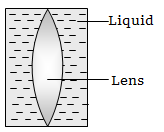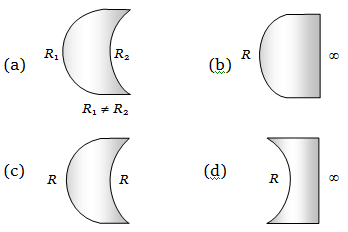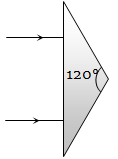Shown in the figure here is a convergent lens placed inside a cell filled with a liquid. The lens has focal length + 20 cm when in air and its material has refractive index 1.50. If the liquid has refractive index 1.60, the focal length of the system is(a) + 80 cm                         (b) – 80 cm

(c) – 24 cm                          (d) –160 cm

Concept Questions :-

Lensmakers' formula
High Yielding Test Series + Question Bank - NEET 2020

Difficulty Level:

Which one of the following spherical lenses does not exhibit dispersion? The radii of curvature of the surfaces of the lenses are as given in the diagramsConcept Questions :-

Lenses
High Yielding Test Series + Question Bank - NEET 2020

Difficulty Level:

The size of the image of an object, which is at infinity, as formed by a convex lens of focal length 30 cm is 2 cm. If a concave lens of focal length 20 cm is placed between the convex lens and the image is at a distance of 26 cm from the convex lens, calculate the new size of the image.

(a) 1.25 cm                              (b) 2.5 cm

(c) 1.05 cm                              (d) 2 cm

Concept Questions :-

Lenses
High Yielding Test Series + Question Bank - NEET 2020

Difficulty Level:

An achromatic prism is made by crown glass prism $\left({A}_{c}=19°\right)$ and flint glass prism $\left({A}_{F}=6°\right)$. If ${}_{C}\mu _{v}=1.5$ and ${}_{F}\mu _{v}=1.66$, then resultant deviation for red coloured ray will be

(a) 1.04°                     (b) 5°

(c) 0.96°                      (d) 13.5°

Concept Questions :-

Prisms
High Yielding Test Series + Question Bank - NEET 2020

Difficulty Level:

An isosceles prism of angle 120° has a refractive index of 1.44. Two parallel monochromatic rays enter the prism parallel to each other in air as shown. The rays emerging from the opposite faces :(a) Are parallel to each other

(b) Are diverging

(c) Make an angle $2{\mathrm{sin}}^{-1}\left(0.72\right)$ with each other

(d) Make an angle $2\left\{{\mathrm{sin}}^{-1}\left(0.72\right)-30°\right\}$ with each other

Concept Questions :-

Prisms
High Yielding Test Series + Question Bank - NEET 2020

Difficulty Level:

A ray of light is incident on the hypotenuse of a right-angled prism after travelling parallel to the base inside the prism. If $\mu$ is the refractive index of the material of the prism, the maximum value of the base angle for which light is totally reflected from the hypotenuse is

(a) ${\mathrm{sin}}^{-1}\left(\frac{1}{\mu }\right)$                             (b) ${\mathrm{tan}}^{-1}\left(\frac{1}{\mu }\right)$

(c) ${\mathrm{sin}}^{-1}\left(\frac{\mu -1}{\mu }\right)$                         (d) ${\mathrm{cos}}^{-1}\left(\frac{1}{\mu }\right)$

Concept Questions :-

Total internal reflection
High Yielding Test Series + Question Bank - NEET 2020

Difficulty Level:

A plano-convex lens when silvered in the plane side behaves like a concave mirror of focal length 30 cm. However, when silvered on the convex side it behaves like a concave mirror of focal length 10 cm. Then the refractive index of its material will be

(a) 3.0                                (b) 2.0

(c) 2.5                                (d) 1.5

Concept Questions :-

Lenses
High Yielding Test Series + Question Bank - NEET 2020

Difficulty Level:

A ray of light travels from an optically denser to rarer medium. The critical angle for the two media is C. The maximum possible deviation of the ray will be

(a) $\left(\frac{\mathrm{\pi }}{2}-C\right)$                          (b) 2C

(c) $\mathrm{\pi }-2\mathrm{C}$                             (d) $\mathrm{\pi }-\mathrm{C}$

Concept Questions :-

Total internal reflection
High Yielding Test Series + Question Bank - NEET 2020

Difficulty Level:

An astronaut is looking down on earth's surface from a space shuttle at an altitude of . Assuming that the astronaut's pupil diameter is 5 mm and the wavelength of visible light is 500 nm. The astronaut will be able to resolve linear object of the size of about

(a) 0.5 m                    (b) 5 m

(c) 50 m                      (d) 500 m

Concept Questions :-

Resolution of Optical devices
High Yielding Test Series + Question Bank - NEET 2020

Difficulty Level:

The average distance between the earth and moon is $38.6×{10}^{4}$ km. The minimum separation between the two points on the surface of the moon that can be resolved by a telescope whose objective lens has a diameter of 5 m with $\lambda =6000\stackrel{\circ }{Α}$ is

(a) 5.65 m                     (b) 28.25 m

(c) 11.30 m                    (d) 56.51 m

Concept Questions :-

Resolution of Optical devices